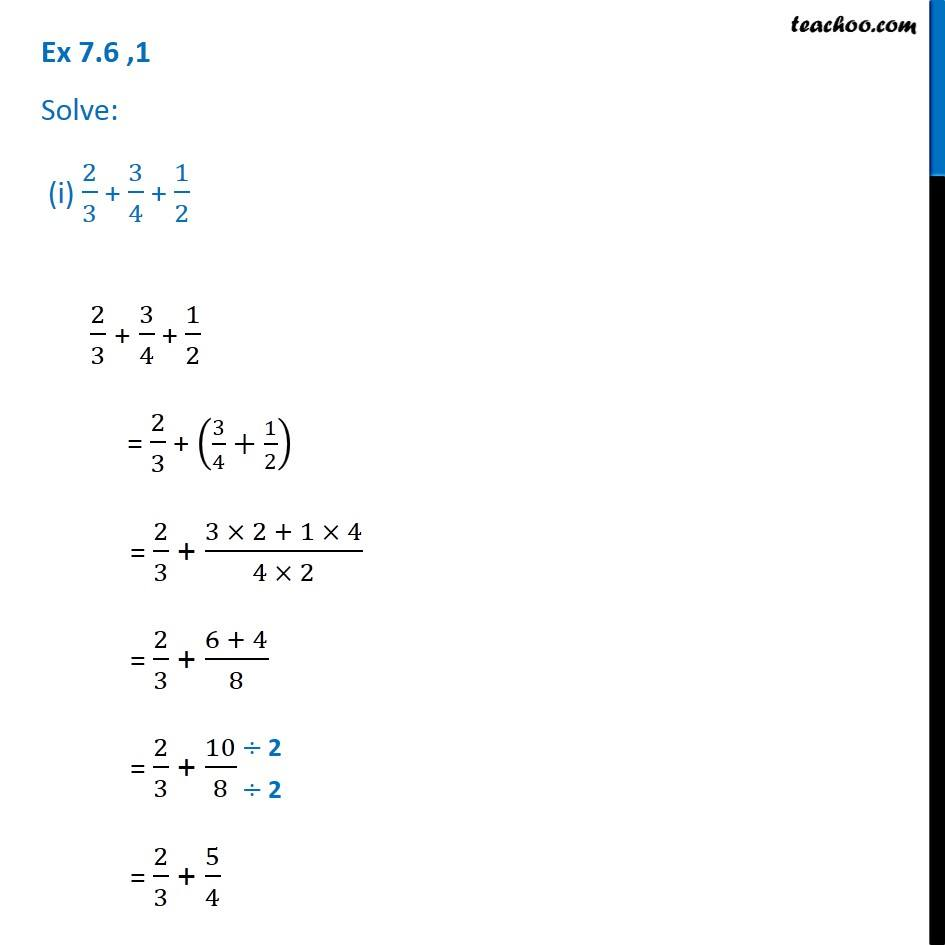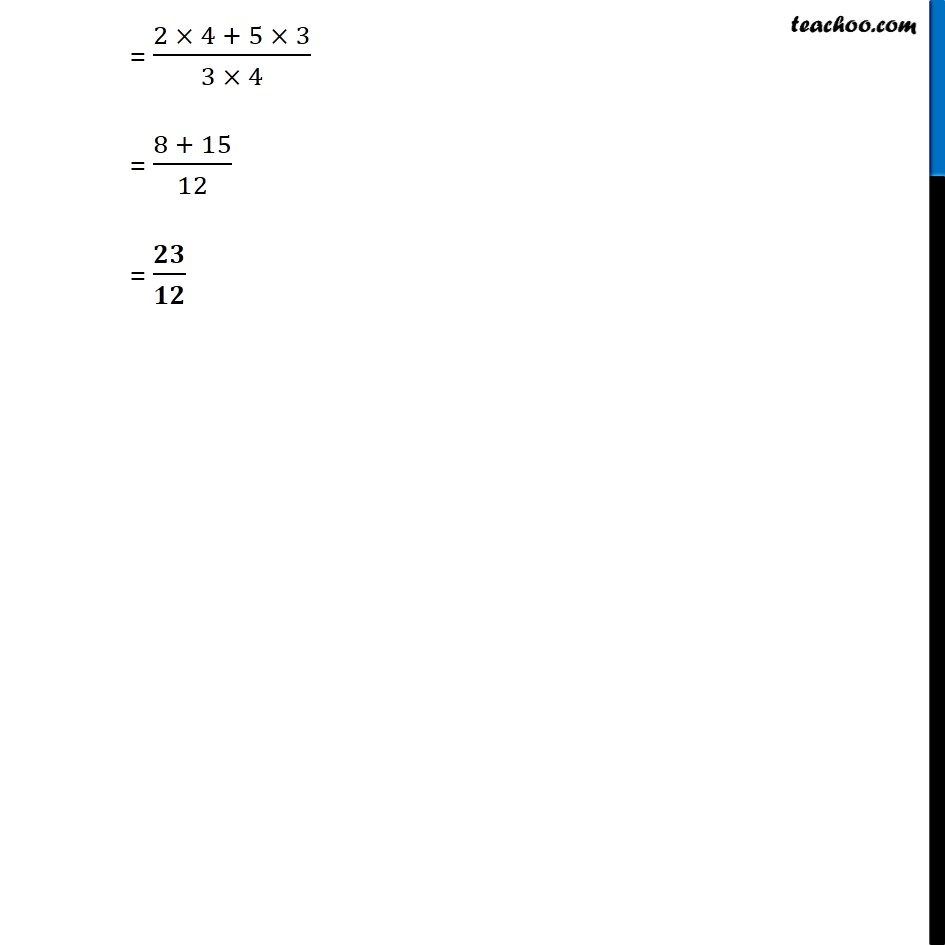1. Chapter 7 Class 6 Fractions
2. Serial order wise
3. Ex 7.6

Transcript

Ex 7.6 ,1 Solve: (i) 2/3 + 3/4 + 1/2 2/3 + 3/4 + 1/2 = 2/3 + (3/4+1/2) = 2/3 + (3 2 + 1 4)/(4 2) = 2/3 + (6 + 4)/8 = 2/3 + 10/8 = 2/3 + 5/4 = (2 4 + 5 3)/(3 4) = (8 + 15)/12 = /